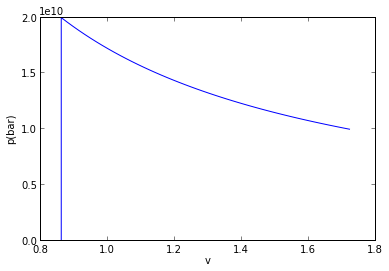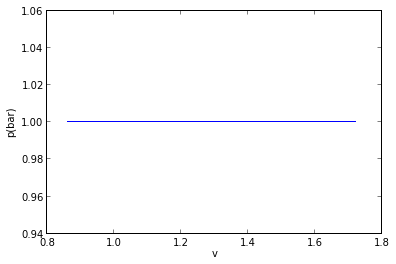# Chapter 3 :- Evaluating Properties¶

## Example 3.1 Page no-80¶

In :
# Given:-
# Those with 1 are of state 1 and 2 are with state 2

# State 1
p1 = 10**5                     # initial pressure in pascal
x1 = 0.5                       # initial quality

T1 = 99.63                     # temperature in degree celcius, from table A-3
v = 0.5                        # volume of container in m3
vf1 = 1.0432*(10**(-3))        # specific volume of fluid in state 1 in m3/Kg(from table A-3)
vg1 = 1.694                    # specific volume of gas in state 1 in m3/kg(from table A-3)

# State 2
p2 = 1.5*(10**5)               # pressure after heating in pascal

T2 = 111.4                     # temperature in degree celcius in state 2, from A-3
vf2 = 1.0582*(10**(-3))        # specific volume of fluid in state 2 in m3/Kg, from A-3
vg2 = 1.159                    # specific volume of gas in state 2 in m3/Kg,from A-3

# Calculations

v1 = vf1 + x1*(vg1-vf1)        # specific volume in state 1 in m3/Kg
v2 = v1                        # specific volume in state 2 in m3/Kg
m = v/v1                       # total mass in Kg
mg1 = x1*m                     # mass of vapour in state 1 in Kg

x2 = (v1-vf2)/(vg2-vf2)        # quality in state 2
mg2 = x2*m                     # mass of vapor in state 2 in Kg

# State 3
p3 = 2.11                      # pressure in state 3 from table A-3

# Results
print '-> The temperature in state 1 is ',round(T1,2),'degree celcius.'
print '-> The temperature in state 2 is ',round(T2,2),'degree celcius.'
print '-> The mass of vapour in state 1 is',round(mg1,2),'kg.'
print '-> The mass of vapour in state 2 is',round(mg2,2),'kg.'
print '-> The pressure corresponding to state 3 is',round(p3,2),'bar.'

-> The temperature in state 1 is  99.63 degree celcius.
-> The temperature in state 2 is  111.4 degree celcius.
-> The mass of vapour in state 1 is 0.29 kg.
-> The mass of vapour in state 2 is 0.43 kg.
-> The pressure corresponding to state 3 is 2.11 bar.

## Example 3.2 Page no-81

In :
# Given:-
m = 0.05                       # mass of ammonia in kg
p1 = 1.5*(10**5)               # initial pressure of ammonia in pascal
v1 = 0.7787                    # specific volume in state 1 in m3/kg from table A-14
v2 = 0.9553                    # specific volume in state 2 in m3/kg from table A-15
T2 = 25.0                      # final temperature in degree celcius

# Calculations

V1 = m*v1                      # volume occupied by ammonia in state 1 in m3
V2 = m*v2                      # volume occupied by ammonia in state 2 in m3
w = (p1*(V2-V1))/1000          # work in KJ

# Results
print '-> The volume occupied by ammonia in state 1 is ',round(V1,2),'m^3.'
print '-> The volume occupied by ammonia in state 2 is ',round(V2,2),'m^3.'
print '-> The work done for the process is',round(w,2),'KJ.'

-> The volume occupied by ammonia in state 1 is  0.04 m^3.
-> The volume occupied by ammonia in state 2 is  0.05 m^3.
-> The work done for the process is 1.32 KJ.

## Example 3.3 Page no-87¶

In :
# Given:-
V = 0.25             # volume of tank in m3
v = 1.673            # specific volume in m3/kg obtained using table A-2

# State 1
T1 = 100.0            # initial temperature in degree celcius
u1 = 2506.5           # specific internal energy in state 1 in KJ/Kg obtained from table A-2

# State 2
p2 = 1.5              # final pressure in bars
T2 = 273.0            # temperature in state 2 in degree celcius obtained from table A-4
u2 = 2767.8           # specific internal energy in state 2 in KJ/Kg obtained from table A-4

# Calculations
m = V/v               # mass of the system in kg
DeltaU = m*(u2-u1)    # change in internal energy in KJ
W = - DeltaU          # from energy balance

# Results
print '-> The temperature at the final state in is',round(T2,2),' degree celcius.'
print '-> The work during the process is',W,'KJ.'

-> The temperature at the final state in is 273.0  degree celcius.
-> The work during the process is -39.0466228332 KJ.

## Example 3.4 Page no-88

In :
# Given:-
# State
P1 = 10*(10**5)                        # initial pressure in pascal
T1 = 400.0                             # initial temperature in degree celcius
v1 = 0.3066                            # specific volume in state 1 in m3/kg obtained from table A-4
u1 = 2957.3                            # specific internal energy in state 1 in KJ/Kg obtained from table A-4

# State 2

v2 = 0.1944                            # specific volume in state 2 in m3/kg obtained from table A-3
w2to3 = 0                              # work in process 2-3

# State 3
v3 = v2
vf3 = 1.0905*(10**(-3))                # specific volume of fluid in state 3 from table A-2
vg3 = 0.3928                           # specific volume of gas in state 3 from table A-2
uf3 = 631.68                           # specific internal energy for fluid in state 3 from table A-2
ug3 = 2559.5                           # specific internal energy for gas in state 3 from table A-2

# Calculations
w1to2 = (P1*(v2-v1))/1000              # work in KJ/Kg in process 1-2
W = w1to2 + w2to3                      # net work in KJ/kg
x3 = (v3-vf3)/(vg3-vf3)
u3 = uf3+x3*(ug3-uf3)                  # specific internal energy in state 3 in Kj/Kg
q = (u3-u1) + W                        # heat transfer in Kj/Kg

# Results
print '-> The work done in the overall process is',W,'KJ/kg.'
print '-> The heat transfer in the overall process is',q,'KJ/kg.'

-> The work done in the overall process is -112.2 KJ/kg.
-> The heat transfer in the overall process is -1486.43658885 KJ/kg.

## Example 3.6 Page no-98

In :
# Given:-
# State 1
p1 = 20.0                      # initial pressure in MPa
T1 = 520.0                     # initial temperature in degree celcius
Z1 = 0.83                      # compressibility factor
R = 8.314                      # universal gas constant in SI unit
n = 1000.0/18.02               # number of moles in a kg of water

# State 2
T2 = 400.0                     # final temperature in degree celcius

# From table A-1
Tc = 647.3                     # critical temperature in kelvin
pc = 22.09                     # critical pressure in MPa

# Calculations
Tr = (T1+273)/Tc               # reduced temperature
Pr = p1/pc                     # reduced pressure
v1 = (Z1*n*R*(T1+273))/(p1*(10**6))
vr = v1*(pc*(10**6))/(n*R*Tc)
Tr2 = (T2+273)/Tc
PR = 0.69                       # at above vr and Tr2
P2 = pc*PR

# Results
print '-> The specific volume in state1 is',v1,'m3/kg and the corresponding value obtained from table A-4 is .01551 m^3/Kg'
print '-> The pressure in MPa in the final state is',P2,' MPa and the corresponding value from the table is 15.16Mpa'

-> The specific volume in state1 is 0.0151836616537 m3/kg and the corresponding value obtained from table A-4 is .01551 m^3/Kg
-> The pressure in MPa in the final state is 15.2421  MPa and the corresponding value from the table is 15.16Mpa

## Example 3.7 Page no:-101

In :
%matplotlib inline

Welcome to pylab, a matplotlib-based Python environment [backend: module://IPython.zmq.pylab.backend_inline].
For more information, type 'help(pylab)'.
In :
# Given:-
T1 = 300.00                                 #temperature in state 1 in kelvin
P1 = 1.00                                   #pressure in state 1 in bar
P2 = 2.00                                   #pressure in state 2 in bar
R = 287.00                                  #gas constant of air in SI units

# Calculations
v1 = (R*T1)/(P1*10**5)                    #specific volume in state 1
v = []
P = []
vv = []
Pa = []
pp = []
pcommon = []
vcommon = []
from numpy import linspace
from pylab import *
P = linspace(1,2,50)
for i in range(0,50):
v.append(i)
v[i] = v1

T2 = (P2*10**5*v1)/R
v3 = (R*T2)/(P1*10**5)
vv = linspace(v1,v3,50)
for i in range(0,50):
Pa.append(i)
Pa[i] = P1

#function[out]= f(inp)
#out = (R*T2)/(inp

VV = linspace(v1,v3,50)
for j in range(0,50):
pp.append(j)
pp[j] = (R*T2)/((VV[j])/(10**5))

vcommon = numpy.concatenate((v, VV))
pcommon = numpy.concatenate((P, pp))

plot(vcommon,pcommon)
xlabel('v')
ylabel('p(bar)')
show()
plot(vv,Pa)
xlabel('v')
ylabel('p(bar)')
show()
#The two steps are shown in one graph and the other on is shown in the other graph"""

print 'The temperature in kelvin in state 2 is T2 = ',T2
print 'The specific volume in state 3 in m^3/kg is  v = ',v3The temperature in kelvin in state 2 is T2 =  600.0
The specific volume in state 3 in m^3/kg is  v =  1.722

## Example 3.8 Page no-108

In :
# Given:-
# State 1
m = 0.9                               # mass of air in kg
T1 = 300.0                            # initial temperature in kelvin
P1 = 1.0                              # initial pressure in bar

# State 2
T2 = 470.0                            # final temperature in kelvin
P2 = 6.0                              # final pressure in bar
Q = -20.0                             # heat transfer in kj

# From table A-22
u1 = 214.07                           # in KJ/kg
u2 = 337.32                           # in KJ/Kg

# Calculations
deltaU = m*(u2-u1)                    # change in internal energy in kj
W = Q - deltaU                        # in KJ/kg

# Results

print '-> The work during the process is ',W,' KJ.'

-> The work during the process is  -130.925  KJ.

## Example 3.9 Page no-109

In :
# Given:-
# State 1
m1 = 2.0                          # initial mass of gas in tank 1 in kg
T1 = 350.0                        # initial temperature in kelvin in tank1
p1 = 0.7                          # initial pressure in bar in tank 1

# State 2
m2 = 8.0                          # initial mass of gas in tank 2 in kg
T2 = 300.0                        # initial temperature in kelvin in tank 2
p2 = 1.2                          # initial pressure in bar in tank 2
Tf = 315.0                        # final equilibrium temperature in kelvin

# From table A-20
Cv = 0.745                        # in KJ/Kg.k

# Calculations
pf = ((m1+m2)*Tf)/((m1*T1/p1)+(m2*T2/p2))
Ui = (m1*Cv*T1)+(m2*Cv*T2)
Uf = (m1+m2)*Cv*Tf
deltaU = Uf-Ui
Q = deltaU

# Results
print '-> The final equilibrium pressure is',pf,'bar.'
print '-> The heat transfer for the process is',Q,'KJ.'

-> The final equilibrium pressure is 1.05 bar.
-> The heat transfer for the process is 37.25 KJ.

## Example 3.11 Page no-113

In :
# Given:-
p1 = 1.0                               # initial pressure in bar
T1 = 295.0                             # initial temperature in kelvin
p2 = 5.0                               # final pressure in bar
n = 1.3                                # polytropic constant
R = 8314/28.97                         # gas constant for air in SI units

# From table A-22
u2 = 306.53
u1 = 210.49

# Calculations
T2 = T1*(p2/p1)**((n-1)/n)
w = R*(T2-T1)/(1-n)
Q = u2-u1+w/1000

# Results
print '-> The work done per unit mass is ',w/1000,'KJ/kg.'
print '-> The heat transfer per unit mass is',Q,'KJ/kg.'

-> The work done per unit mass is  -126.928201465 KJ/kg.
-> The heat transfer per unit mass is -30.888201465 KJ/kg.